Since I migrated my blog from Github Pages to blogdown and Netlify, I wanted to start migrating (most of) my old posts too - and use that opportunity to update them and make sure the code still works.

Here I am updating my very first machine learning post from 27 Nov 2016: Can we predict flu deaths with Machine Learning and R?. Changes are marked as bold comments.

The main changes I made are:

• using the `tidyverse` more consistently throughout the analysis
• focusing on comparing multiple imputations from the `mice` package, rather than comparing different algorithms
• using `purrr`, `map()`, `nest()` and `unnest()` to model and predict the machine learning algorithm over the different imputed datasets

Among the many nice R packages containing data collections is the outbreaks package. It contains a dataset on epidemics and among them is data from the 2013 outbreak of influenza A H7N9 in China as analysed by Kucharski et al. (2014):

A. Kucharski, H. Mills, A. Pinsent, C. Fraser, M. Van Kerkhove, C. A. Donnelly, and S. Riley. 2014. Distinguishing between reservoir exposure and human-to-human transmission for emerging pathogens using case onset data. PLOS Currents Outbreaks. Mar 7, edition 1. doi: 10.1371/currents.outbreaks.e1473d9bfc99d080ca242139a06c455f.

A. Kucharski, H. Mills, A. Pinsent, C. Fraser, M. Van Kerkhove, C. A. Donnelly, and S. Riley. 2014. Data from: Distinguishing between reservoir exposure and human-to-human transmission for emerging pathogens using case onset data. Dryad Digital Repository. http://dx.doi.org/10.5061/dryad.2g43n.

I will be using their data as an example to show how to use Machine Learning algorithms for predicting disease outcome.

``````library(outbreaks)
library(tidyverse)
library(plyr)
library(mice)
library(caret)
library(purrr)``````

# The data

The dataset contains case ID, date of onset, date of hospitalization, date of outcome, gender, age, province and of course outcome: Death or Recovery.

## Pre-processing

Change: variable names (i.e. column names) have been renamed, dots have been replaced with underscores, letters are all lower case now.

Change: I am using the tidyverse notation more consistently.

First, I’m doing some preprocessing, including:

• renaming missing data as NA
• setting column types
• gathering date columns
• changing factor names of dates (to make them look nicer in plots) and of `province` (to combine provinces with few cases)
``````fluH7N9_china_2013\$age[which(fluH7N9_china_2013\$age == "?")] <- NA
fluH7N9_china_2013_gather <- fluH7N9_china_2013 %>%
mutate(case_id = paste("case", case_id, sep = "_"),
age = as.numeric(age)) %>%
gather(Group, Date, date_of_onset:date_of_outcome) %>%
mutate(Group = as.factor(mapvalues(Group, from = c("date_of_onset", "date_of_hospitalisation", "date_of_outcome"),
to = c("date of onset", "date of hospitalisation", "date of outcome"))),
province = mapvalues(province, from = c("Anhui", "Beijing", "Fujian", "Guangdong", "Hebei", "Henan", "Hunan", "Jiangxi", "Shandong", "Taiwan"), to = rep("Other", 10)))``````

I’m also

• adding a third gender level for unknown gender
``````levels(fluH7N9_china_2013_gather\$gender) <- c(levels(fluH7N9_china_2013_gather\$gender), "unknown")
fluH7N9_china_2013_gather\$gender[is.na(fluH7N9_china_2013_gather\$gender)] <- "unknown"
``````##   case_id outcome gender age province         Group       Date
## 1  case_1   Death      m  58 Shanghai date of onset 2013-02-19
## 2  case_2   Death      m   7 Shanghai date of onset 2013-02-27
## 3  case_3   Death      f  11    Other date of onset 2013-03-09
## 4  case_4    <NA>      f  18  Jiangsu date of onset 2013-03-19
## 5  case_5 Recover      f  20  Jiangsu date of onset 2013-03-19
## 6  case_6   Death      f   9  Jiangsu date of onset 2013-03-21``````

For plotting, I am defining a custom `ggplot2` theme:

``````my_theme <- function(base_size = 12, base_family = "sans"){
theme_minimal(base_size = base_size, base_family = base_family) +
theme(
axis.text = element_text(size = 12),
axis.text.x = element_text(angle = 45, vjust = 0.5, hjust = 0.5),
axis.title = element_text(size = 14),
panel.grid.major = element_line(color = "grey"),
panel.grid.minor = element_blank(),
panel.background = element_rect(fill = "aliceblue"),
strip.background = element_rect(fill = "lightgrey", color = "grey", size = 1),
strip.text = element_text(face = "bold", size = 12, color = "black"),
legend.position = "bottom",
legend.justification = "top",
legend.box = "horizontal",
legend.box.background = element_rect(colour = "grey50"),
legend.background = element_blank(),
panel.border = element_rect(color = "grey", fill = NA, size = 0.5)
)
}``````

And use that theme to visualize the data:

``````ggplot(data = fluH7N9_china_2013_gather, aes(x = Date, y = age, fill = outcome)) +
stat_density2d(aes(alpha = ..level..), geom = "polygon") +
geom_jitter(aes(color = outcome, shape = gender), size = 1.5) +
geom_rug(aes(color = outcome)) +
scale_y_continuous(limits = c(0, 90)) +
labs(
fill = "Outcome",
color = "Outcome",
alpha = "Level",
shape = "Gender",
x = "Date in 2013",
y = "Age",
title = "2013 Influenza A H7N9 cases in China",
subtitle = "Dataset from 'outbreaks' package (Kucharski et al. 2014)",
caption = ""
) +
facet_grid(Group ~ province) +
my_theme() +
scale_shape_manual(values = c(15, 16, 17)) +
scale_color_brewer(palette="Set1", na.value = "grey50") +
scale_fill_brewer(palette="Set1")``````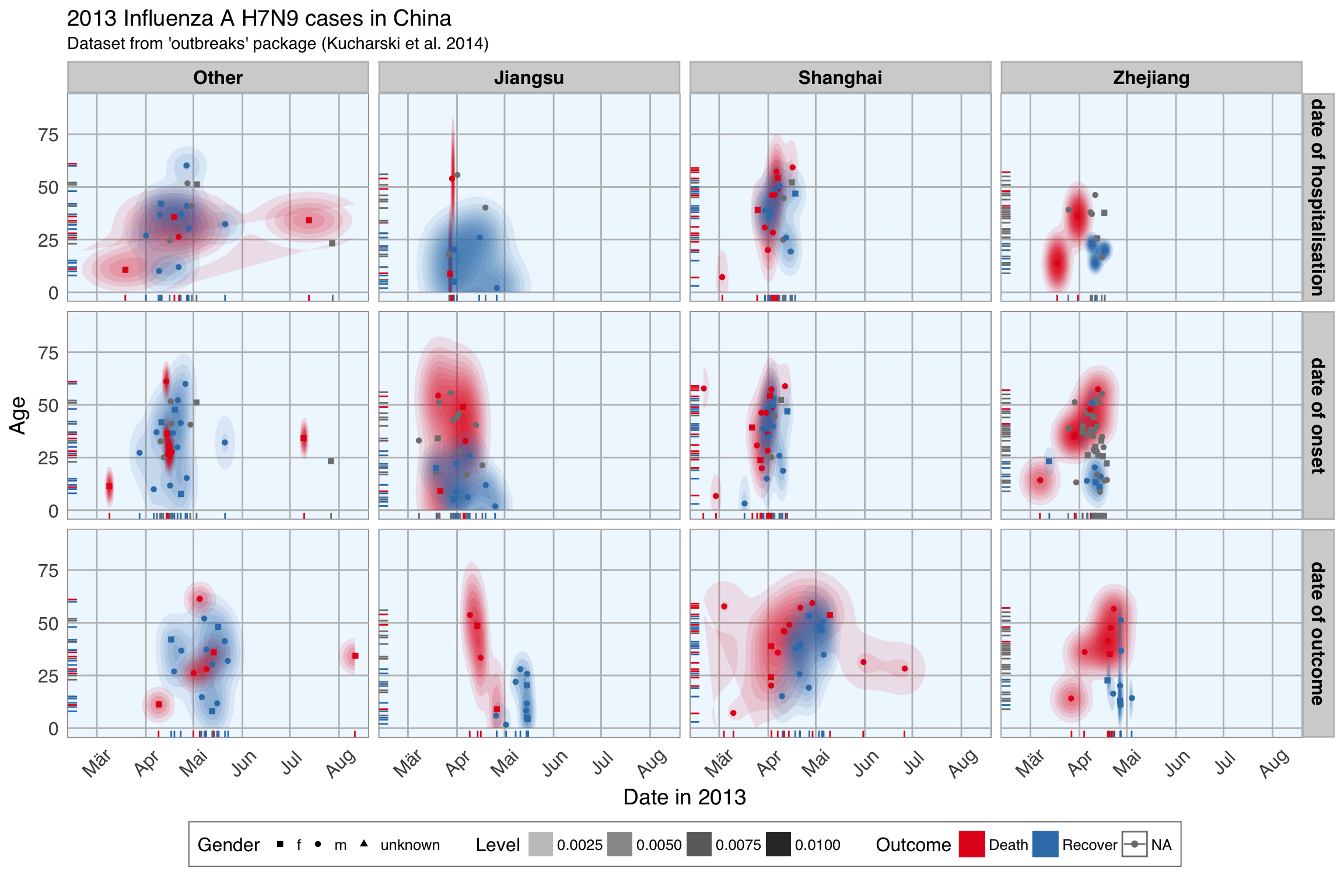``````ggplot(data = fluH7N9_china_2013_gather, aes(x = Date, y = age, color = outcome)) +
geom_point(aes(color = outcome, shape = gender), size = 1.5, alpha = 0.6) +
geom_path(aes(group = case_id)) +
facet_wrap( ~ province, ncol = 2) +
my_theme() +
scale_shape_manual(values = c(15, 16, 17)) +
scale_color_brewer(palette="Set1", na.value = "grey50") +
scale_fill_brewer(palette="Set1") +
labs(
color = "Outcome",
shape = "Gender",
x = "Date in 2013",
y = "Age",
title = "2013 Influenza A H7N9 cases in China",
subtitle = "Dataset from 'outbreaks' package (Kucharski et al. 2014)",
caption = "\nTime from onset of flu to outcome."
)``````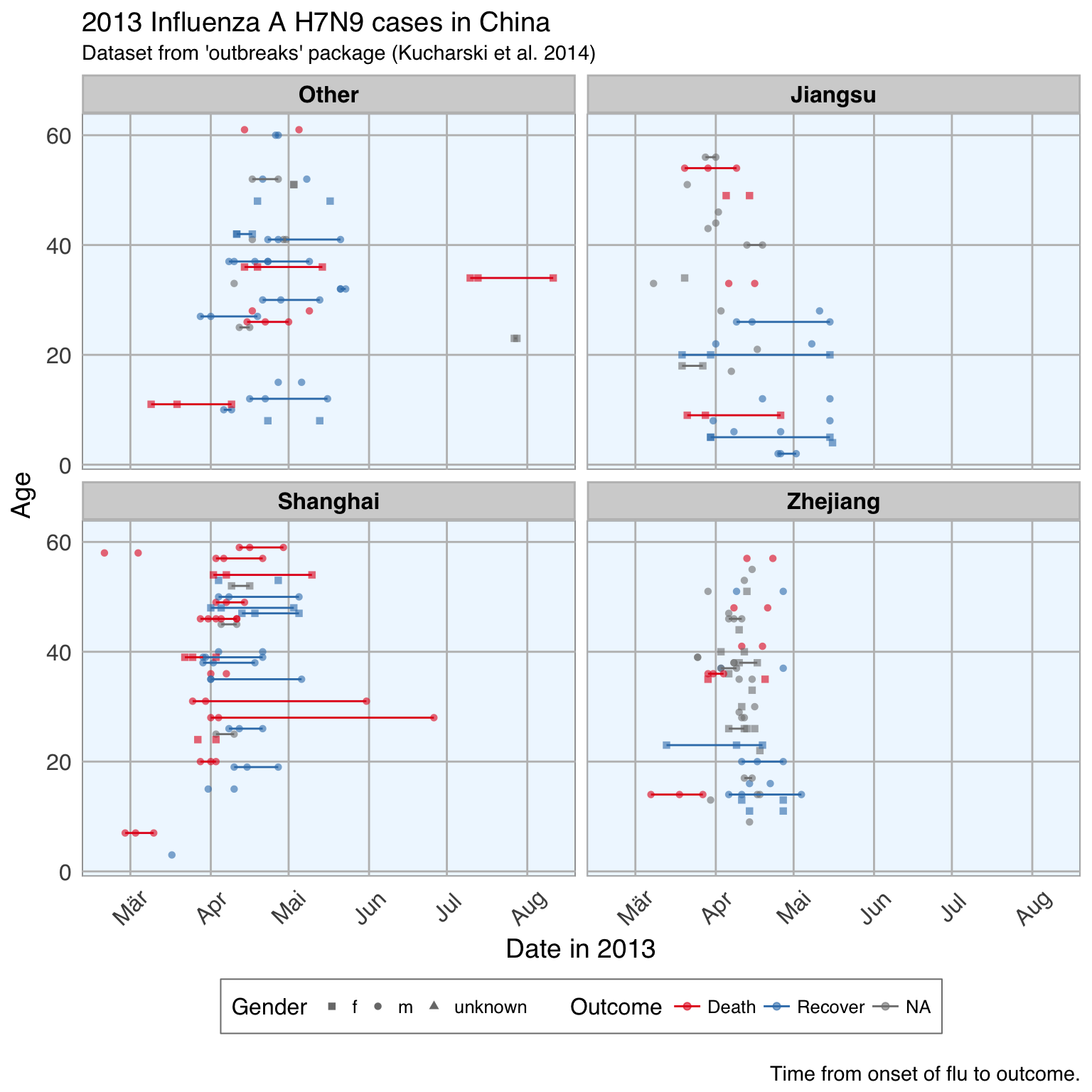# Features

In machine learning-speak features are what we call the variables used for model training. Using the right features dramatically influences the accuracy and success of your model.

For this example, I am keeping age, but I am also generating new features from the date information and converting gender and province into numerical values.

``````dataset <- fluH7N9_china_2013 %>%
mutate(hospital = as.factor(ifelse(is.na(date_of_hospitalisation), 0, 1)),
gender_f = as.factor(ifelse(gender == "f", 1, 0)),
province_Jiangsu = as.factor(ifelse(province == "Jiangsu", 1, 0)),
province_Shanghai = as.factor(ifelse(province == "Shanghai", 1, 0)),
province_Zhejiang = as.factor(ifelse(province == "Zhejiang", 1, 0)),
province_other = as.factor(ifelse(province == "Zhejiang" | province == "Jiangsu" | province == "Shanghai", 0, 1)),
days_onset_to_outcome = as.numeric(as.character(gsub(" days", "",
as.Date(as.character(date_of_outcome), format = "%Y-%m-%d") -
as.Date(as.character(date_of_onset), format = "%Y-%m-%d")))),
days_onset_to_hospital = as.numeric(as.character(gsub(" days", "",
as.Date(as.character(date_of_hospitalisation), format = "%Y-%m-%d") -
as.Date(as.character(date_of_onset), format = "%Y-%m-%d")))),
age = age,
early_onset = as.factor(ifelse(date_of_onset < summary(fluH7N9_china_2013\$date_of_onset)[], 1, 0)),
early_outcome = as.factor(ifelse(date_of_outcome < summary(fluH7N9_china_2013\$date_of_outcome)[], 1, 0))) %>%
subset(select = -c(2:4, 6, 8))
rownames(dataset) <- dataset\$case_id
dataset[, -2] <- as.numeric(as.matrix(dataset[, -2]))
``````##   case_id outcome age hospital gender_f province_Jiangsu province_Shanghai
## 1       1   Death  87        0        0                0                 1
## 2       2   Death  27        1        0                0                 1
## 3       3   Death  35        1        1                0                 0
## 4       4    <NA>  45        1        1                1                 0
## 5       5 Recover  48        1        1                1                 0
## 6       6   Death  32        1        1                1                 0
##   province_Zhejiang province_other days_onset_to_outcome
## 1                 0              0                    13
## 2                 0              0                    11
## 3                 0              1                    31
## 4                 0              0                    NA
## 5                 0              0                    57
## 6                 0              0                    36
##   days_onset_to_hospital early_onset early_outcome
## 1                     NA           1             1
## 2                      4           1             1
## 3                     10           1             1
## 4                      8           1            NA
## 5                     11           1             0
## 6                      7           1             1``````
``summary(dataset\$outcome)``
``````##   Death Recover    NA's
##      32      47      57``````

## Imputing missing values

I am using the `mice` package for imputing missing values

Note: Since publishing this blogpost I learned that the idea behind using `mice` is to compare different imputations to see how stable they are, instead of picking one imputed set as fixed for the remainder of the analysis. Therefore, I changed the focus of this post a little bit: in the old post I compared many different algorithms and their outcome; in this updated version I am only showing the Random Forest algorithm and focus on comparing the different imputed datasets. I am ignoring feature importance and feature plots because nothing changed compared to the old post.

• `md.pattern()` shows the pattern of missingness in the data:
``md.pattern(dataset)``
``````##    case_id hospital province_Jiangsu province_Shanghai province_Zhejiang
## 42       1        1                1                 1                 1
## 27       1        1                1                 1                 1
##  2       1        1                1                 1                 1
##  2       1        1                1                 1                 1
## 18       1        1                1                 1                 1
##  1       1        1                1                 1                 1
## 36       1        1                1                 1                 1
##  3       1        1                1                 1                 1
##  3       1        1                1                 1                 1
##  2       1        1                1                 1                 1
##          0        0                0                 0                 0
##    province_other age gender_f early_onset outcome early_outcome
## 42              1   1        1           1       1             1
## 27              1   1        1           1       1             1
##  2              1   1        1           1       1             0
##  2              1   1        1           0       1             1
## 18              1   1        1           1       0             0
##  1              1   1        1           1       1             0
## 36              1   1        1           1       0             0
##  3              1   1        1           0       1             0
##  3              1   1        1           0       0             0
##  2              1   0        0           0       1             0
##                 0   2        2          10      57            65
##    days_onset_to_outcome days_onset_to_hospital
## 42                     1                      1   0
## 27                     1                      0   1
##  2                     0                      1   2
##  2                     0                      0   3
## 18                     0                      1   3
##  1                     0                      0   3
## 36                     0                      0   4
##  3                     0                      0   4
##  3                     0                      0   5
##  2                     0                      0   6
##                       67                     74 277``````
• `mice()` generates the imputations
``dataset_impute <- mice(data = dataset[, -2],  print = FALSE)``
• by default, `mice()` calculates five (m = 5) imputed data sets
• we can combine them all in one output with the `complete("long")` function
• I did not want to impute missing values in the `outcome` column, so I have to merge it back in with the imputed data
``````datasets_complete <- right_join(dataset[, c(1, 2)],
complete(dataset_impute, "long"),
by = "case_id") %>%
select(-.id)``````
``head(datasets_complete)``
``````##   case_id outcome .imp age hospital gender_f province_Jiangsu
## 1       1   Death    1  87        0        0                0
## 2       2   Death    1  27        1        0                0
## 3       3   Death    1  35        1        1                0
## 4       4    <NA>    1  45        1        1                1
## 5       5 Recover    1  48        1        1                1
## 6       6   Death    1  32        1        1                1
##   province_Shanghai province_Zhejiang province_other days_onset_to_outcome
## 1                 1                 0              0                    13
## 2                 1                 0              0                    11
## 3                 0                 0              1                    31
## 4                 0                 0              0                    28
## 5                 0                 0              0                    57
## 6                 0                 0              0                    36
##   days_onset_to_hospital early_onset early_outcome
## 1                      5           1             1
## 2                      4           1             1
## 3                     10           1             1
## 4                      8           1             1
## 5                     11           1             0
## 6                      7           1             1``````

Let’s compare the distributions of the five different imputed datasets:

``````datasets_complete %>%
gather(x, y, age:early_outcome) %>%
ggplot(aes(x = y, fill = .imp, color = .imp)) +
facet_wrap(~ x, ncol = 3, scales = "free") +
geom_density(alpha = 0.4) +
scale_fill_brewer(palette="Set1", na.value = "grey50") +
scale_color_brewer(palette="Set1", na.value = "grey50") +
my_theme()``````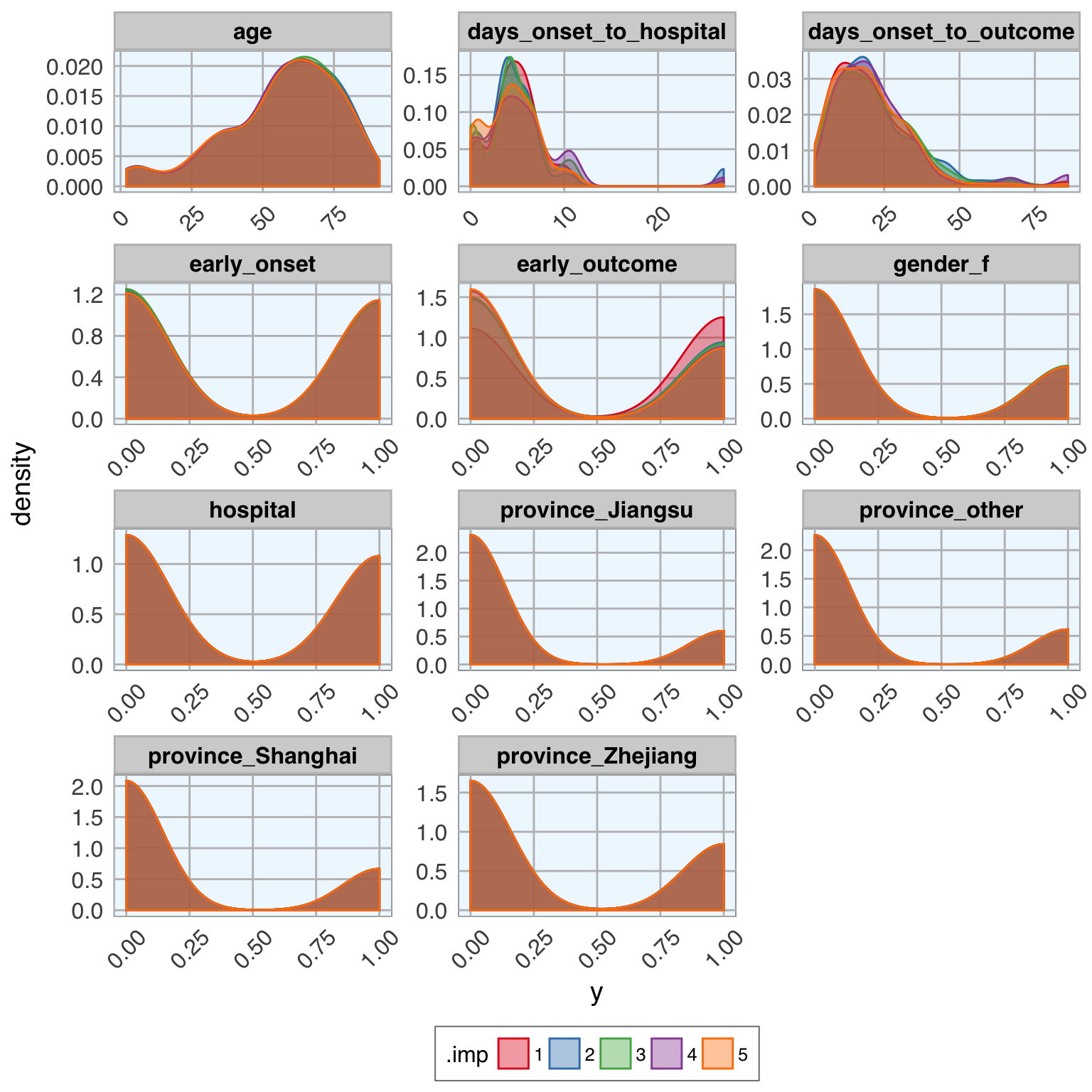# Test, train and validation data sets

Now, we can go ahead with machine learning!

The dataset contains a few missing values in the `outcome` column; those will be the test set used for final predictions (see the old blog post for this).

``````train_index <- which(is.na(datasets_complete\$outcome))
train_data <- datasets_complete[-train_index, ]
test_data  <- datasets_complete[train_index, -2]``````

The remainder of the data will be used for modeling. Here, I am splitting the data into 70% training and 30% test data.

Because I want to model each imputed dataset separately, I am using the `nest()` and `map()` functions.

``````set.seed(42)
val_data <- train_data %>%
group_by(.imp) %>%
nest() %>%
mutate(val_index = map(data, ~ createDataPartition(.\$outcome, p = 0.7, list = FALSE)),
val_train_data = map2(data, val_index, ~ .x[.y, ]),
val_test_data = map2(data, val_index, ~ .x[-.y, ]))``````

# Machine Learning algorithms

## Random Forest

To make the code tidier, I am first defining the modeling function with the parameters I want.

``````model_function <- function(df) {
caret::train(outcome ~ .,
data = df,
method = "rf",
preProcess = c("scale", "center"),
trControl = trainControl(method = "repeatedcv", number = 5, repeats = 3, verboseIter = FALSE))
}``````

Next, I am using the nested tibble from before to `map()` the model function, predict the outcome and calculate confusion matrices.

``````set.seed(42)
val_data_model <- val_data %>%
mutate(model = map(val_train_data, ~ model_function(.x)),
predict = map2(model, val_test_data, ~ data.frame(prediction = predict(.x, .y[, -2]))),
predict_prob = map2(model, val_test_data, ~ data.frame(outcome = .y[, 2],
prediction = predict(.x, .y[, -2], type = "prob"))),
confusion_matrix = map2(val_test_data, predict, ~ confusionMatrix(.x\$outcome, .y\$prediction)),
confusion_matrix_tbl = map(confusion_matrix, ~ as.tibble(.x\$table)))``````

## Comparing accuracy of models

To compare how the different imputations did, I am plotting

• the confusion matrices:
``````val_data_model %>%
unnest(confusion_matrix_tbl) %>%
ggplot(aes(x = Prediction, y = Reference, fill = n)) +
facet_wrap(~ .imp, ncol = 5, scales = "free") +
geom_tile() +
my_theme()``````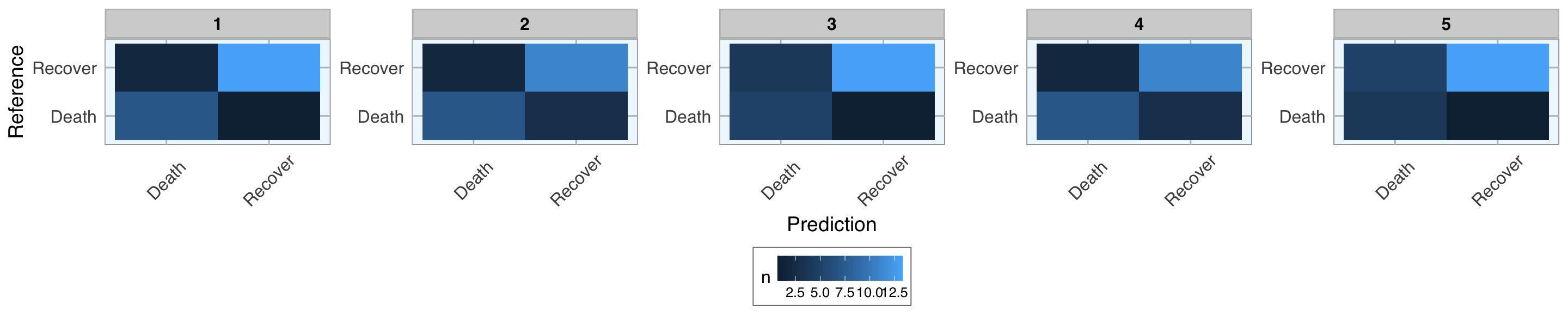• and the prediction probabilities for correct and wrong predictions:
``````val_data_model %>%
unnest(predict_prob) %>%
gather(x, y, prediction.Death:prediction.Recover) %>%
ggplot(aes(x = x, y = y, fill = outcome)) +
facet_wrap(~ .imp, ncol = 5, scales = "free") +
geom_boxplot() +
scale_fill_brewer(palette="Set1", na.value = "grey50") +
my_theme()``````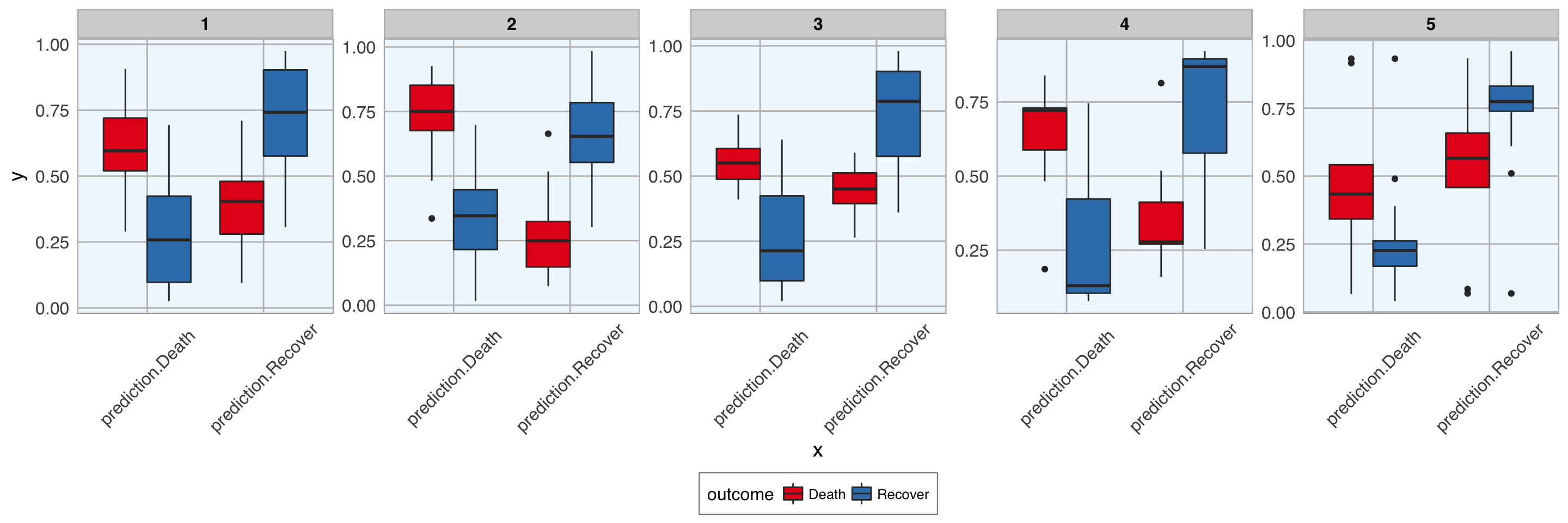Hope, you found that example interesting and helpful!

``sessionInfo()``
``````## R version 3.5.0 (2018-04-23)
## Platform: x86_64-apple-darwin15.6.0 (64-bit)
## Running under: macOS High Sierra 10.13.4
##
## Matrix products: default
## BLAS: /Library/Frameworks/R.framework/Versions/3.5/Resources/lib/libRblas.0.dylib
## LAPACK: /Library/Frameworks/R.framework/Versions/3.5/Resources/lib/libRlapack.dylib
##
## locale:
##  de_DE.UTF-8/de_DE.UTF-8/de_DE.UTF-8/C/de_DE.UTF-8/de_DE.UTF-8
##
## attached base packages:
##  stats     graphics  grDevices utils     datasets  methods   base
##
## other attached packages:
##   bindrcpp_0.2.2  caret_6.0-79    mice_2.46.0     lattice_0.20-35
##   plyr_1.8.4      forcats_0.3.0   stringr_1.3.1   dplyr_0.7.4
##   purrr_0.2.4     readr_1.1.1     tidyr_0.8.0     tibble_1.4.2
##  ggplot2_2.2.1   tidyverse_1.2.1 outbreaks_1.3.0
##
## loaded via a namespace (and not attached):
##   nlme_3.1-137        lubridate_1.7.4     dimRed_0.1.0
##   RColorBrewer_1.1-2  httr_1.3.1          rprojroot_1.3-2
##   tools_3.5.0         backports_1.1.2     R6_2.2.2
##  rpart_4.1-13        lazyeval_0.2.1      colorspace_1.3-2
##  nnet_7.3-12         withr_2.1.2         tidyselect_0.2.4
##  mnormt_1.5-5        compiler_3.5.0      cli_1.0.0
##  rvest_0.3.2         xml2_1.2.0          labeling_0.3
##  bookdown_0.7        scales_0.5.0        sfsmisc_1.1-2
##  DEoptimR_1.0-8      psych_1.8.4         robustbase_0.93-0
##  randomForest_4.6-14 digest_0.6.15       foreign_0.8-70
##  rmarkdown_1.9       pkgconfig_2.0.1     htmltools_0.3.6
##  rstudioapi_0.7      bindr_0.1.1         jsonlite_1.5
##  ModelMetrics_1.1.0  magrittr_1.5        Matrix_1.2-14
##  Rcpp_0.12.17        munsell_0.4.3       abind_1.4-5
##  stringi_1.2.2       yaml_2.1.19         MASS_7.3-50
##  recipes_0.1.2       grid_3.5.0          parallel_3.5.0
##  crayon_1.3.4        haven_1.1.1         splines_3.5.0
##  hms_0.4.2           knitr_1.20          pillar_1.2.2
##  reshape2_1.4.3      codetools_0.2-15    stats4_3.5.0
##  CVST_0.2-1          magic_1.5-8         glue_1.2.0
##  evaluate_0.10.1     blogdown_0.6        modelr_0.1.2
##  foreach_1.4.4       cellranger_1.1.0    gtable_0.2.0
##  kernlab_0.9-26      assertthat_0.2.0    DRR_0.0.3
##  xfun_0.1            gower_0.1.2         prodlim_2018.04.18
##  broom_0.4.4         e1071_1.6-8         class_7.3-14
##  survival_2.42-3     geometry_0.3-6      timeDate_3043.102
##  RcppRoll_0.2.2      iterators_1.0.9     lava_1.6.1
##  ipred_0.9-6``````